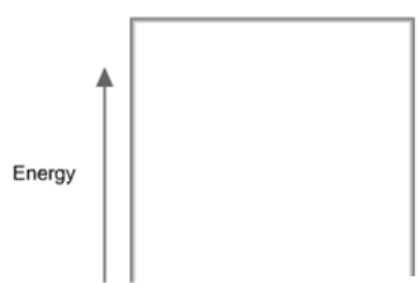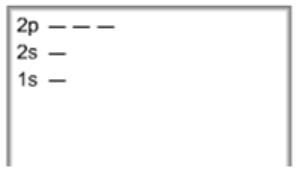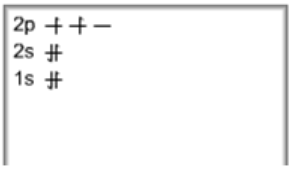# Problem: Construct the orbital diagram for As.HintStart by adding the appropriate subshells. For example, carbon is in the 2p block of the periodic table, and so you need to show the 2p subshell and everything below it.Next, click the orbitals to add electrons (represented as arrows). For carbon, you would need to show a total of six electrons.

###### FREE Expert Solution

We’re being asked to construct the orbital diagram for As. For that, we first need to determine the electron configuration of As.

Recall that for a neutral element, Atomic number = # of protons = # of electrons

The atomic number of As is 33 and since it’s a neutral element, this means As has 33 electrons.###### Problem Details

Construct the orbital diagram for As.Hint

Start by adding the appropriate subshells. For example, carbon is in the 2p block of the periodic table, and so you need to show the 2p subshell and everything below it.Next, click the orbitals to add electrons (represented as arrows). For carbon, you would need to show a total of six electrons.